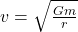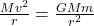Question

Find the orbital speed v of a satellite in a circular orbit of radius r around a planet of mass m.

1.Answer:Explanation:

Given

Orbital speed is v

Mass of planet is m

Radius of circular orbit is r

suppose M is the mas of satellite then centripetal force on satellite is equal to the Gravitational Pull.where G=gravitational constant

thus on solving we get